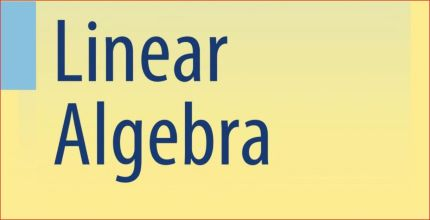Linear Algebra for UPSC

Ramanasri IAS Maths Optional is teaching Linear Algebra for UPSC. Ramanasri Sir is teaching all the 13 Modules. For example from 1 st Module Linear Algebra to Last i.e., 13th Module Mechanics & Fluid Dynamics. This Linear Algebra Module is Medium Level and In that Matrices, Part is very easy like Vector Analysis, Complex Analysis, Linear Programming,  Numerical Analysis, and Computer Programming, Calculus,

Medium Level Modules: Ordinary Differential Equations, Partial Differential Equations, Analytical Geometry.
Difficult Level Modules: Mechanics and Fluid Dynamics, Statics and Dynamics, Modern Algebra, Real Analysis.

In this Below we are giving Linear Algebra for UPSC Maths Syllabus. And also Linear Algebra for IFS or IFoS Maths Syllabus.

Linear Algebra for UPSC Maths Syllabus | IAS Maths Syllabus

Vector spaces over R and C, linear dependence and independence, subspaces, bases, dimension; Linear transformations, rank and nullity, a matrix of a linear transformation. Algebra of Matrices; Row and column reduction, Echelon form, congruence’s and similarity; Rank of a matrix; Inverse of a matrix; Solution of system of linear equations; Eigenvalues and Eigenvectors, characteristic polynomial, Cayley-Hamilton theorem, Symmetric, skew-symmetric, Hermitian, Skew-Hermitian, orthogonal and unitary matrices, and their Eigenvalues.

Linear Algebra for IFS Maths Syllabus | IFoS Maths Syllabus.

Vector, space, linear dependence, and independence, subspaces, bases, dimensions. Finite-dimensional vector spaces. Matrices, Cayley-Hamilton theorem, Eigenvalues, and Eigenvectors, the matrix of a linear transformation, row and column reduction, Echelon form, equivalence, congruence and similarity, reduction to canonical form, rank, orthogonal, symmetrical, skew symmetrical, unitary, Hermitian, skew-Hermitian forms their Eigenvalues. Orthogonal and unitary reduction of quadratic and Hermitian forms, positive definite quadratic forms.

Linear Algebra Common topics for UPSC Maths Syllabus | IAS Maths Syllabus & IFS or IFoS Maths Syllabus

Vector spaces over R and C, linear dependence and independence, subspaces, bases, dimension; matrix of a linear transformation. Algebra of Matrices; Row and column reduction, Echelon form, congruence’s and similarity; Rank of a matrix; Inverse of a matrix; Solution of system of linear equations; Eigenvalues and Eigenvectors, characteristic polynomial, Cayley-Hamilton theorem, Symmetric, skew-symmetric, Hermitian, Skew-Hermitian, orthogonal and unitary matrices, and their Eigenvalues.

List of topics of Linear Algebra for ONLY UPSC Maths Syllabus | IAS Maths Syllabus

Linear transformations, rank and nullity,

List of topics of Linear Algebra for ONLY IFS Maths Syllabus | IFoS Maths Syllabus

Finite-dimensional vector spaces. reduction to canonical form. Orthogonal and unitary reduction of quadratic and Hermitian forms, positive definite quadratic forms.

Click on this below links to know more details